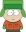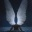Coffeehouse for desis
Would you like to react to this message? Create an account in a few clicks or log in to continue.

# Middle School Math Quiz

3 posters##Middle School Math Quiz

I selected these 4 relatively easy questions from an actual quiz of 30 problems published in a math magazine. I also have the answers for these questions (with explanations) given in that magazine.

1. Each day Jane makes an open-faced sandwich using only one slice of bread. She uses 1 kind of meat or 1 kind of cheese or 1 kind of each. If she chooses from 4 kinds of bread, 5 kinds of meat, and 3 kinds of cheese, how many different sandwiches can she make?

2. Ten boys and girls write their names on slips of paper – one name per slip – to enter a prize drawing. Two of the names are drawn at random without replacement. If the probability that both winners are boys is 1/15, how many boys are in the group?

3. If Jack and Jill each randomly choose a whole number from 1 to 1000 inclusive, what is the probability that Jill’s number is larger than Jack’s?

4. Mental math – neither calculator nor pencil permitted. The sum of the first 50 positive odd integersis 50-squared. Find the sum of the first 50 positive even integers.

indophile

Posts : 4338
Join date : 2011-04-29
Location : Glenn Dale, MD

##Re: Middle School Math Quiz

1. 4x5 + 4x3 + 4x3x5 = 92charvaka

Posts : 4347
Join date : 2011-04-28
Location : Berkeley, CA

##Re: Middle School Math Quiz

2. 3 boysHellsangel

Posts : 14721
Join date : 2011-04-28

##Re: Middle School Math Quiz

2. 3. Basically the denominator of the probability should be 9x10. Expressing it as a fraction of 90, we have 6/90. We also need teh numerator to be (n - 1) x n where n is the original number of boys. So n = 3.charvaka

Posts : 4347
Join date : 2011-04-28
Location : Berkeley, CA

##Re: Middle School Math Quiz

4. 50-squared + 50 = 2550. Basically each of the 50 even integers is 1 more than the corresponding odd number in the first series.charvaka

Posts : 4347
Join date : 2011-04-28
Location : Berkeley, CA

##Re: Middle School Math Quiz

3. My intuitive answer is 1/2. Neither of them have higher probability of picking the large number.charvaka

Posts : 4347
Join date : 2011-04-28
Location : Berkeley, CA

##Re: Middle School Math Quiz

Incorrect.

indophile

Posts : 4338
Join date : 2011-04-29
Location : Glenn Dale, MD

##Re: Middle School Math Quiz

indophile wrote:Incorrect.
Which one? Or all of them?charvaka

Posts : 4347
Join date : 2011-04-28
Location : Berkeley, CA

##Re: Middle School Math Quiz

charvaka wrote:
indophile wrote:Incorrect.
Which one? Or all of them?

Yours and HA's answers to 1,2,and 4 are correct.

indophile

Posts : 4338
Join date : 2011-04-29
Location : Glenn Dale, MD

##Middle School Math Quiz - ANSWERS

indophile wrote:

1. 92. Jane can make 4*5 = 20 kinds of meat sandwiches, 4*3=12 kinds of cheese sandwiches, and 4*5*3 = 60 kinds of meat and cheese sandwiches. Thus, she can make a total of 20+12+60 = 92 different types of sandwiches.

2. 3 boys. Let b= the number of boys. The probability that the first winner is a boy is b/10, and the conditional probability that the second winner is a boy is (b-1)/9. The probability that both events occur is is the product of these two probabilities: b(b-1)/(10*9) = 1/15. In order for b > 0, b = 3. There are 3 boys in the group.

3. 999/2000. By symmetry, the probability that Jill’s number exceeds Jack’s number equals the probability that Jack’s number exceeds Jill’s number. Therefore, 2*P(Jill’s number > Jack’s number) + P (Jill’s number = Jack’s number) = 1. The probability that Jack and Jill independently choose the same number is 1/1000. Then, (1- 1/1000)/2 = 999/2000.

4. 2550. Sum the first 100 integers using Gauss method (Sum = n(n+1)/2). It’s 5050. Then subtract the sum of the first 50 odd integers: 5050-2500 = 2550 which is the sum of the first 50 even integers.
(Charvaka's solution is even easier than this)

indophile

Posts : 4338
Join date : 2011-04-29
Location : Glenn Dale, MD

##Re: Middle School Math Quiz

Very nice... I had the idea that neither of them has an "edge," but didn't take it to the logical conclusion.charvaka

Posts : 4347
Join date : 2011-04-28
Location : Berkeley, CA

##Re: Middle School Math Quiz